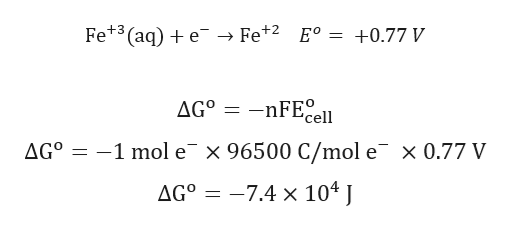# You are given the following three half-reactions: (1) Fe3+(aq) + e−→Fe2+(aq) Eo  = +0.77 V   (2) Fe2+(aq) + 2e−→Fe(s) Eo  = −0.44 V   (3) Fe3+(aq) + 3e−  →  Fe(s)  Part 1 out of 3 (a) CalculateΔGo  for (1) and (2) from their Eo  values. ΔGo  for (1) = ×10 J Enter your answer in scientific notation. ΔGo  for (2) = ×10 J Enter your answer in scientific notation.

Question
64 views
You are given the following three half-reactions:

(1) Fe3+(aq) + e
Fe2+(aq)

E
 o
= +0.77 V

(2) Fe2+(aq) + 2e
Fe(s)

E
 o
= −0.44 V

(3) Fe3+(aq) + 3e
Fe(s)

 Part 1 out of 3
(a) Calculate
Δ
G
 o
for (1) and (2) from their E
 o
values.

Δ
G
 o
for (1) =

×
10

J

Δ
G
 o
for (2) =

×
10

J

check_circle

Step 1

Calculation for ΔGo fo...help_outlineImage TranscriptioncloseFe+3 (aq) + e → Fe+2 E° = +0.77 V AGº -nFE cell AG° = -1 mol e¯ x 96500 C/mol e x 0.77 V AG° = –7.4 x 104 J fullscreen

### Want to see the full answer?

See Solution

#### Want to see this answer and more?

Solutions are written by subject experts who are available 24/7. Questions are typically answered within 1 hour.*

See Solution
*Response times may vary by subject and question.
Tagged in

### Chemistry